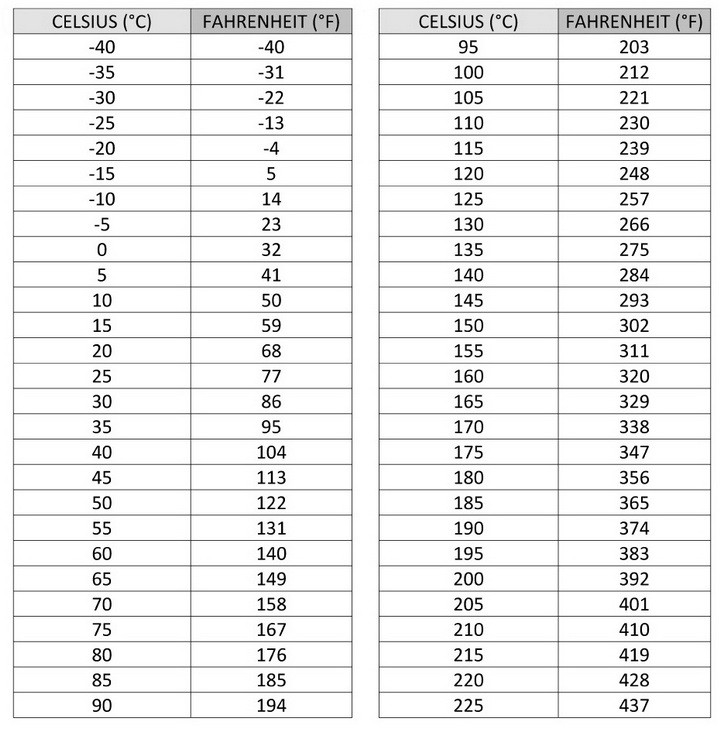# How To Convert Celsius (°C) to Fahrenheit (°F) Degrees With Chart

How to convert Celsius to Fahrenheit. At times when you are in a different country or have an appliance that is made or assembled in Europe, the temp controls are in Celsius. Here is how to easily (with a C to F calculator) find out how to convert Celsius to Fahrenheit or vice versa.

There are two main temperature scales:
°C, the Celsius Scale (the Metric System used in most other countries)
°F, the Fahrenheit Scale (used in the USA)

Conversion, definition and formulas for Celsius and Fahrenheit calculationsCelsius to Fahrenheit conversion chart

Celsius to Fahrenheit Conversion video

You can convert Fahrenheit to Celsius or vice versa by using simple addition, subtraction, multiplication, and division.

Lets start with 0 degrees Celsius, this equals 32 degrees Fahrenheit, so…
0 °C = 32 °F

The temperature T in degrees Fahrenheit (°F) is equal to the temperature T in degrees Celsius (°C) times 1.8 plus 32…
T(°F) = T(°C) × 9/5 + 32
OR
T(°F) = T(°C) × 1.8 + 32

An example would be:
Convert degrees in Celsius to degrees in Fahrenheit:
Temperature (°Fahrenheit) = 35°C × 1.8 + 32 = 95°F
OR
Temperature (°Fahrenheit) = 25°C × 1.8 + 32 = 77°F

If you need help with converting F to C or C to F, let us know by leaving a comment below and we will assist.